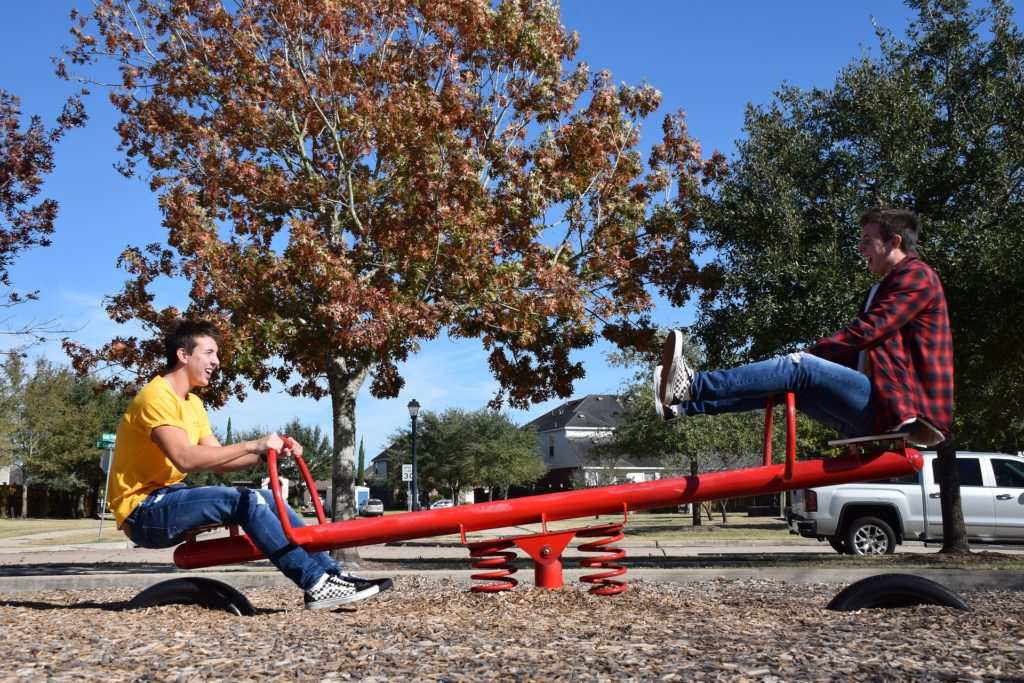# What are moments?#### Get straight to the facts about the science of pivots and levers.

Background

Moments come into play when forces act on an object that has a fixed point. For example, turning a door handle, sitting on a seesaw or closing a pair of scissors. When forces are applied to these objects they rotate around their fixed point, also known as the pivot or fulcrum. The ‘moment’ is the turning effect of the force. It tells us how much the object will rotate and in which direction. Put simply, a moment is a twist. It is also known as torque.

In brief

To calculate a moment you need to know two things: the force (which is measured in Newtons) and the perpendicular distance between the pivot to the line of action of the force (which is measured in metres). When you multiply these two numbers you get the moment, which is measured in Newton metres (Nm).

For example, a seesaw has a pivot at the centre. If a person sits on one end, the moment can be calculated by taking the force of their weight on the seat and multiplying it by the distance from the seat to the middle of the seesaw.

Moments also have a direction, either clockwise or anticlockwise. When no one is sitting on the seesaw, the moments in both directions are equal. But when one person sits down the seesaw moves. If another person joins them by sitting on the other end, their body weight creates a moment in the opposite direction.

Moment (Nm) = Force (N) x distance (m)

Summary

Moments are the turning effects of forces. They have a direction, either clockwise or anticlockwise, and they can be calculated by multiplying the force exerted by its distance from the pivot.

#### Moments in action

Take a trip to your local park to test turning moments for yourself

Everyday moments

Moments are everywhere. Trying to undo a bolt with your fingers is almost impossible, but add a spanner and suddenly it becomes very easy to turn. This is because you’re increasing the distance between the force and the pivot and therefore you’re increasing the turning moment.

The same principle applies when using a screwdriver to pry open a can of syrup or paint, or closing the handles of a pair of scissors to slice through a sheet of card or a piece of string. The further away you apply the force from the pivot, the easier the task will become.

Moments don’t have to be on opposite sides of the pivot, either. A heavy load in a wheelbarrow is close to the wheel, while the handles are further away. This means that you need less force in order to lift the contents. Understanding the simple principles of moments makes everyday tasks an awful lot easier to perform.